# 卷积（Convolution）¶

## 一、卷积提出背景¶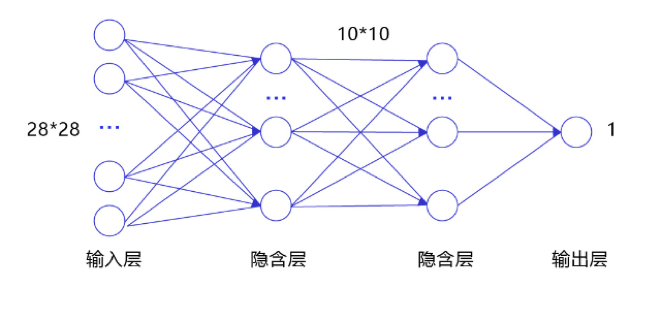1. 输入数据的空间信息被丢失。 空间上相邻的像素点往往具有相似的RGB值，RGB的各个通道之间的数据通常密切相关，但是转化成1维向量时，这些信息被丢失。如 图2 所示，空间位置相邻的两个点A和B，转化成1维向量后并没有体现出他们之间的空间关联性。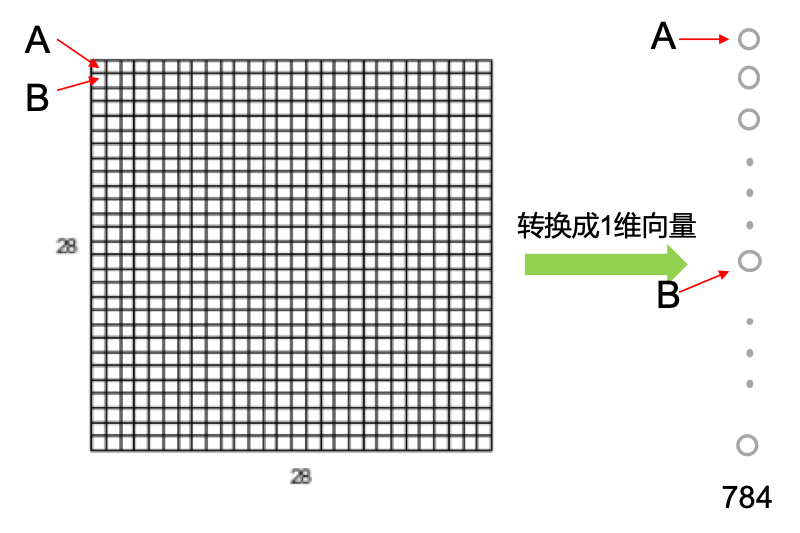2. 模型参数过多，容易发生过拟合。 由于每个像素点都要跟所有输出的神经元相连接。当图片尺寸变大时，输入神经元的个数会按图片尺寸的平方增大，导致模型参数过多，容易发生过拟合。例如：对于一幅$$1000\times 1000$$ 的输入图像而言，如果下一个隐含层的神经元数目为$$10^6$$ 个，那么将会有$$1000\times 1000\times 10^6=10^{12}$$ 个权重参数，可以想象，如此大规模的参数量使得网络很难训练。

## 二、卷积核 / 特征图 / 卷积计算¶

### 应用示例¶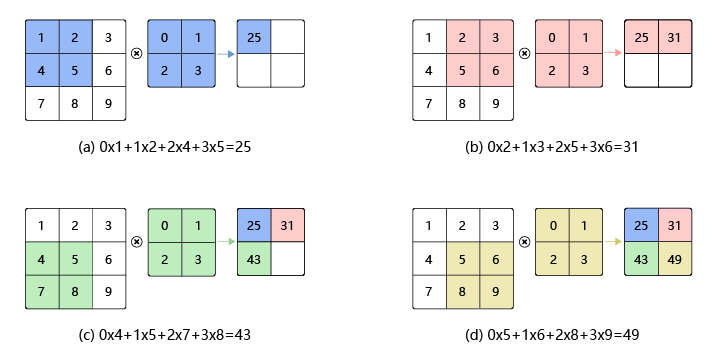• 如图3（a）所示：左边的图大小是$$3\times3$$，表示输入数据是一个维度为$$3\times3$$的二维数组；中间的图大小是$$2\times2$$，表示一个维度为$$2\times2$$的二维数组，我们将这个二维数组称为卷积核。先将卷积核的左上角与输入数据的左上角（即：输入数据的(0, 0)位置）对齐，把卷积核的每个元素跟其位置对应的输入数据中的元素相乘，再把所有乘积相加，得到卷积输出的第一个结果：

$0\times1 + 1\times2 + 2\times4 + 3\times5 = 25 \ \ \ \ \ \ \ (a)$
• 如图3（b）所示：将卷积核向右滑动，让卷积核左上角与输入数据中的(0,1)位置对齐，同样将卷积核的每个元素跟其位置对应的输入数据中的元素相乘，再把这4个乘积相加，得到卷积输出的第二个结果：

$0\times2 + 1\times3 + 2\times5 + 3\times6 = 31 \ \ \ \ \ \ \ (b)$
• 如图3（c）所示：将卷积核向下滑动，让卷积核左上角与输入数据中的(1, 0)位置对齐，可以计算得到卷积输出的第三个结果：

$0\times4 + 1\times5 + 2\times7 + 3\times8 = 43 \ \ \ \ \ \ \ (c)$
• 如图3（d）所示：将卷积核向右滑动，让卷积核左上角与输入数据中的(1, 1)位置对齐，可以计算得到卷积输出的第四个结果：

$0\times5 + 1\times6 + 2\times8 + 3\times9 = 49 \ \ \ \ \ \ \ (d)$

$b[i, j] = \sum_{u,v}{a[i+u, j+v]\cdot w[u, v]}$

$b[i, j] = a[i+0, j+0]\cdot w[0, 0] + a[i+0, j+1]\cdot w[0, 1] + a[i+1, j+0]\cdot w[1, 0] + a[i+1, j+1]\cdot w[1, 1]$

$0\times1 + 1\times2 + 2\times4 + 3\times5 \mathbf{\ + 1} = 26$
$0\times2 + 1\times3 + 2\times5 + 3\times6 \mathbf{\ + 1} = 32$
$0\times4 + 1\times5 + 2\times7 + 3\times8 \mathbf{\ + 1} = 44$
$0\times5 + 1\times6 + 2\times8 + 3\times9 \mathbf{\ + 1} = 50$

1. 输入图像的边缘像素因其处于边缘位置，因此无法参与卷积计算；

2. 图像卷积计算实质上是对图像进行了下采样 (down sampling)操作。对卷积得到的图像结果不断卷积滤波，则原始图像就会被“层层抽象、层层约减”，从而使得蕴涵在图像中的重要信息“显山露水”；

3. 卷积核中的权重系数$$w_i$$是通过数据驱动机制学习得到，其用来捕获图像中某像素点及其邻域像素点所构成的特有空间模式。一旦从数据中学习得到权重系数，这些权重系数就刻画了图像中像素点构成的空间分布不同模式。

### 应用示例¶

$H_{out} = H - k_h + 1$
$W_{out} = W - k_w + 1$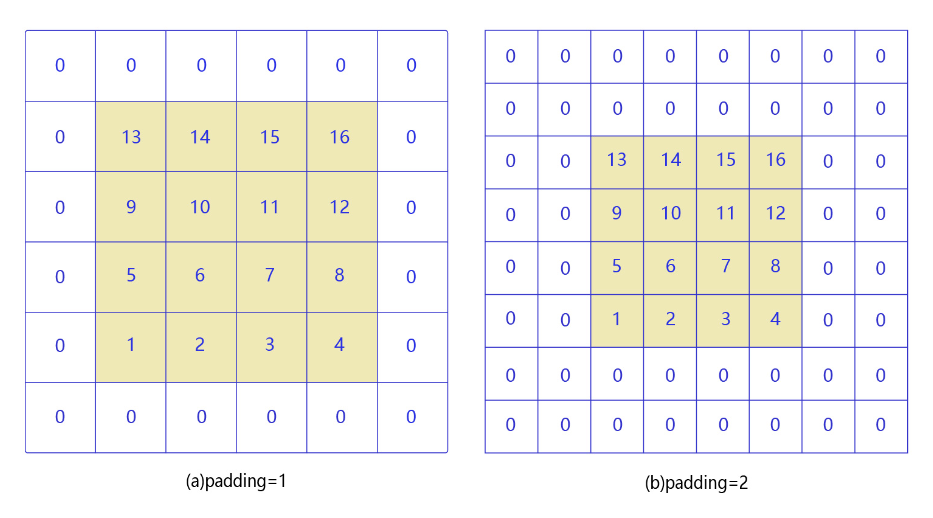• 如图4（a）所示：填充的大小为1，填充值为0。填充之后，输入图片尺寸从$$4\times4$$变成了$$6\times6$$，使用$$3\times3$$的卷积核，输出图片尺寸为$$4\times4$$

• 如图4（b）所示：填充的大小为2，填充值为0。填充之后，输入图片尺寸从$$4\times4$$变成了$$8\times8$$，使用$$3\times3$$的卷积核，输出图片尺寸为$$6\times6$$

$H_{out} = H + p_{h1} + p_{h2} - k_h + 1$
$W_{out} = W + p_{w1} + p_{w2} - k_w + 1$

$H_{out} = H + 2p_h - k_h + 1$
$W_{out} = W + 2p_w - k_w + 1$

## 四、步长（Stride）¶

### 应用示例¶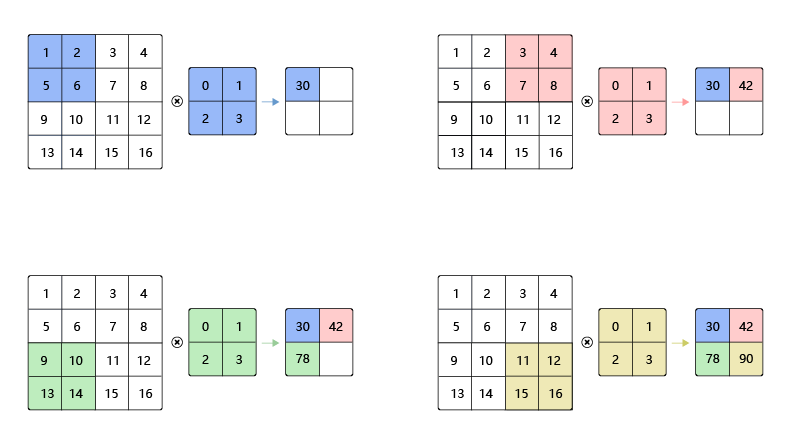$H_{out} = \frac{H + 2p_h - k_h}{s_h} + 1$
$W_{out} = \frac{W + 2p_w - k_w}{s_w} + 1$

$H_{out} = \frac{100 + 2 - 3}{2} + 1 = 50$
$W_{out} = \frac{100 + 2 - 3}{2} + 1 = 50$

## 五、感受野（Receptive Field）¶

### 应用示例¶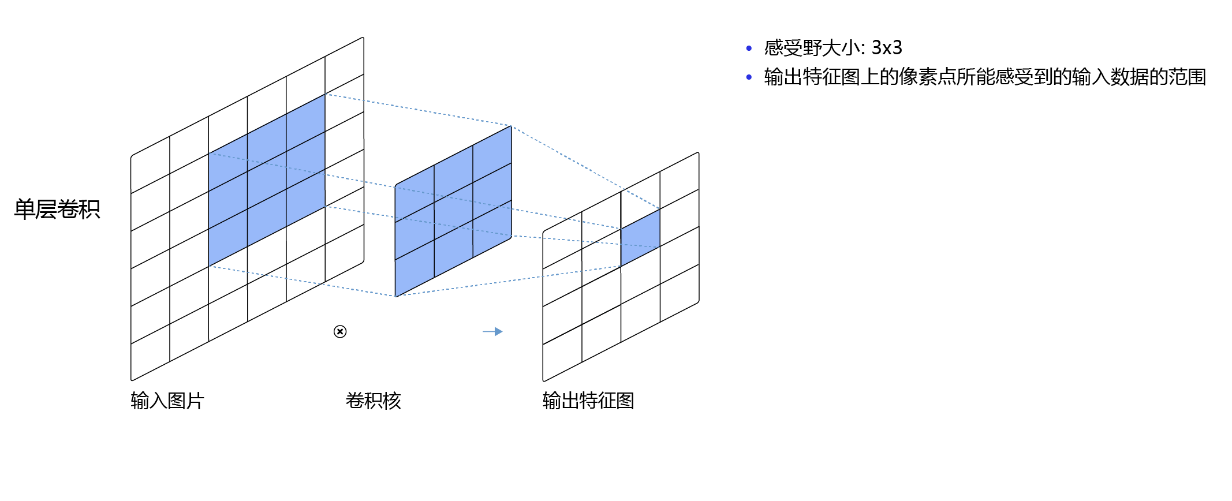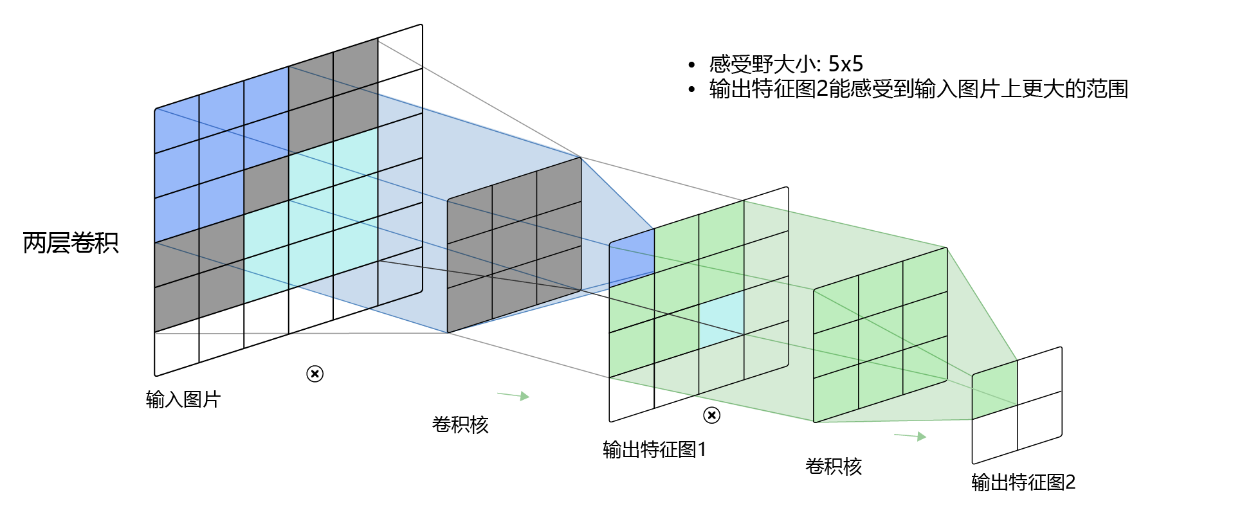## 六、多输入通道、多输出通道和批量操作¶

### 多输入通道场景¶

1. 对每个通道分别设计一个2维数组作为卷积核，卷积核数组的形状是$$C_{in}\times{k_h}\times{k_w}$$

2. 对任一通道$$C_{in} \in [0, C_{in})$$，分别用大小为$$k_h\times{k_w}$$的卷积核在大小为$$H_{in}\times{W_{in}}$$的二维数组上做卷积。

3. 将这$$C_{in}$$个通道的计算结果相加，得到的是一个形状为$$H_{out}\times{W_{out}}$$的二维数组。

### 应用示例¶

1. 对每个通道分别设计一个2维数组作为卷积核，卷积核数组的形状是$$3\times{k_h}\times{k_w}$$

2. 对任一通道$$c_{in} \in [0, 3)$$，分别用大小为$$k_h\times{k_w}$$的卷积核在大小为$$H_{in}\times{W_{in}}$$的二维数组上做卷积。

3. 将这$$3$$个通道的计算结果相加，得到的是一个形状为$$H_{out}\times{W_{out}}$$的二维数组。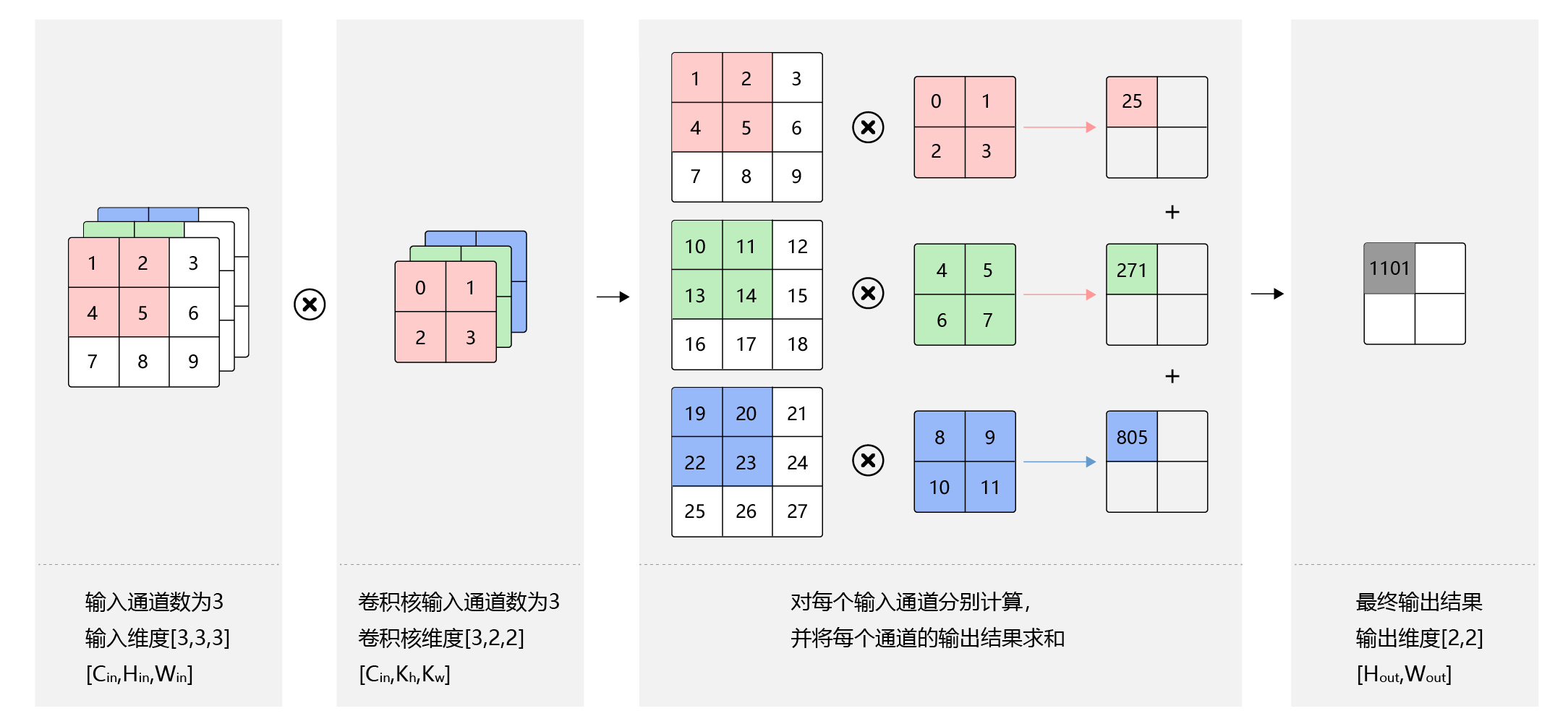### 多输出通道场景¶

1. 对任一输出通道$$c_{out} \in [0, C_{out})$$，分别使用上面描述的形状为$$C_{in}\times{k_h}\times{k_w}$$的卷积核对输入图片做卷积。

2. 将这$$C_{out}$$个形状为$$H_{out}\times{W_{out}}$$的二维数组拼接在一起，形成维度为$$C_{out}\times{H_{out}}\times{W_{out}}$$的三维数组。

### 应用示例¶

1. 对任一输出通道$$c_{out} \in [0, 2)$$，分别使用上面描述的形状为$$3\times{k_h}\times{k_w}$$的卷积核对输入图片做卷积。

2. 将这$$2$$个形状为$$H_{out}\times{W_{out}}$$的二维数组拼接在一起，形成维度为$$2\times{H_{out}}\times{W_{out}}$$的三维数组。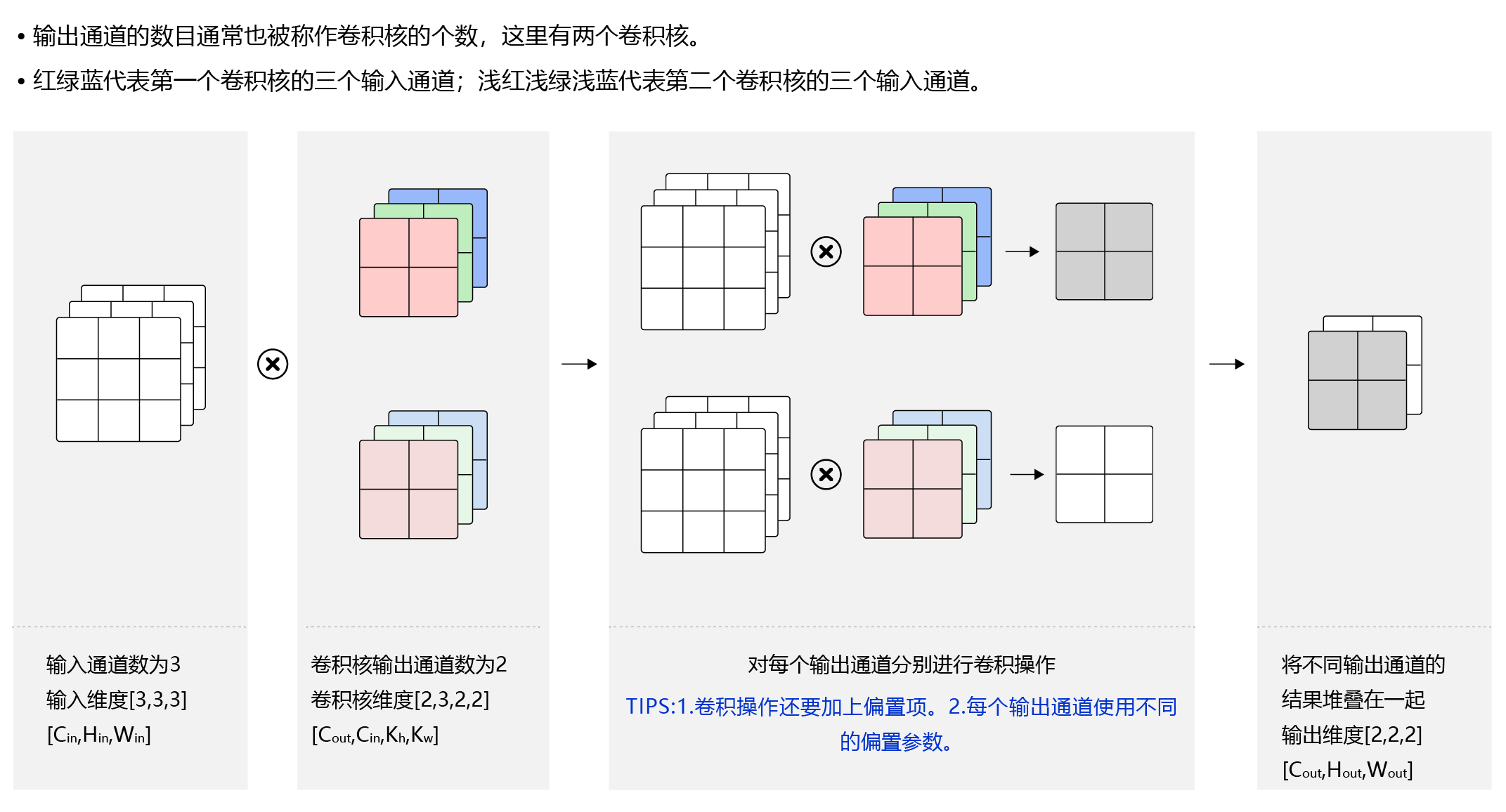### 应用示例¶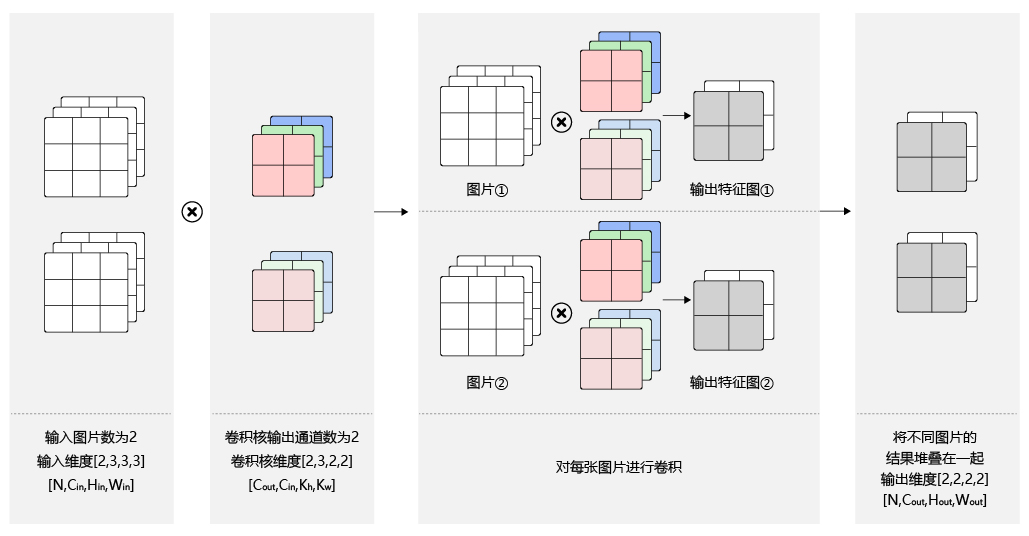## 七、卷积优势¶

• 保留空间信息

• 局部连接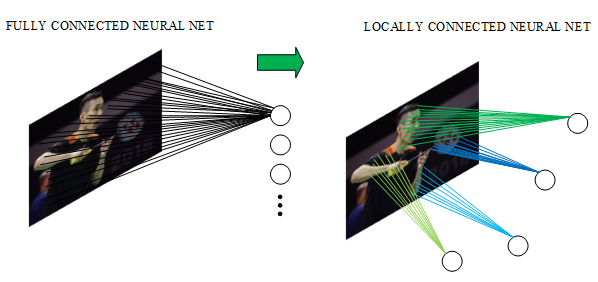• 权重共享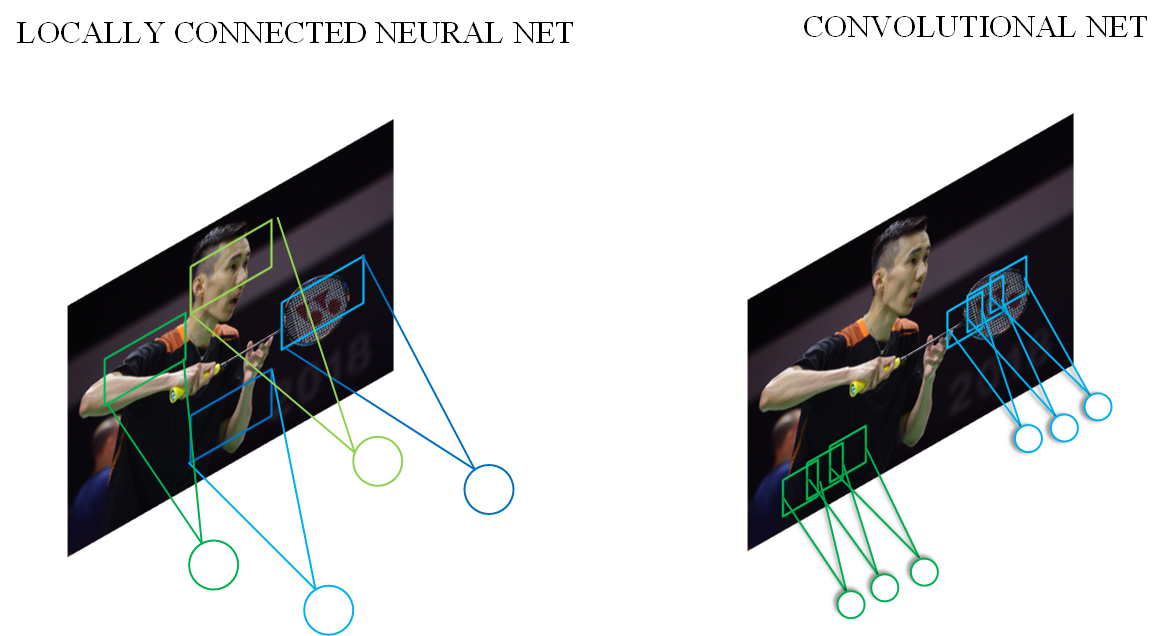• 不同层级卷积提取不同特征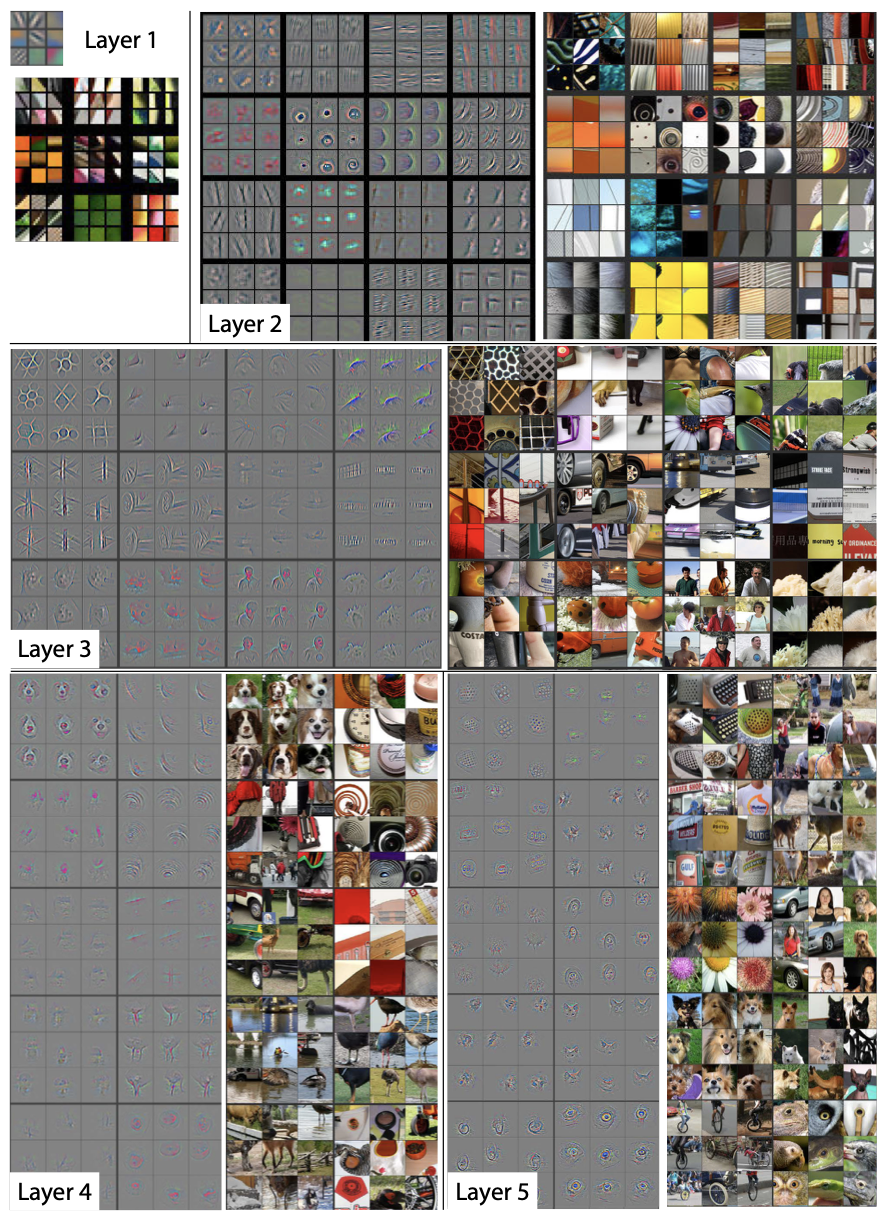## 八、卷积应用示例¶

### 案例1：简单的黑白边界检测¶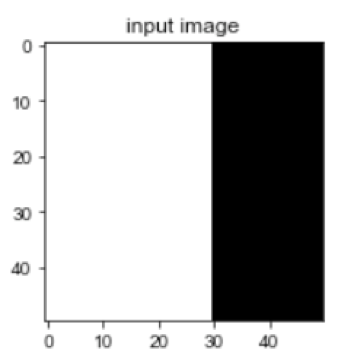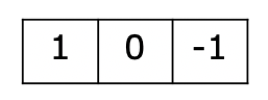import matplotlib.pyplot as plt
import numpy as np
%matplotlib inline

# 创建初始化权重参数w
w = np.array([1, 0, -1], dtype='float32')
# 将权重参数调整成维度为[cout, cin, kh, kw]的四维张量
w = w.reshape([1, 1, 1, 3])
# 创建卷积算子，设置输出通道数，卷积核大小，和初始化权重参数
# kernel_size = [1, 3]表示kh = 1, kw=3
# 创建卷积算子的时候，通过参数属性weight_attr指定参数初始化方式
# 这里的初始化方式时，从numpy.ndarray初始化卷积参数
conv = Conv2D(in_channels=1, out_channels=1, kernel_size=[1, 3],
initializer=Assign(value=w)))

# 创建输入图片，图片左边的像素点取值为1，右边的像素点取值为0
img = np.ones([50,50], dtype='float32')
img[:, 30:] = 0.
# 将图片形状调整为[N, C, H, W]的形式
x = img.reshape([1,1,50,50])
# 使用卷积算子作用在输入图片上
y = conv(x)
# 将输出tensor转化为numpy.ndarray
out = y.numpy()
f = plt.subplot(121)
f.set_title('input image', fontsize=15)
plt.imshow(img, cmap='gray')
f = plt.subplot(122)
f.set_title('output featuremap', fontsize=15)
# 卷积算子Conv2D输出数据形状为[N, C, H, W]形式
# 此处N, C=1，输出数据形状为[1, 1, H, W]，是4维数组
# 但是画图函数plt.imshow画灰度图时，只接受2维数组
# 通过numpy.squeeze函数将大小为1的维度消除
plt.imshow(out.squeeze(), cmap='gray')
plt.show()

# 查看卷积层的权重参数名字和数值
print(conv.weight)
# 参看卷积层的偏置参数名字和数值
print(conv.bias)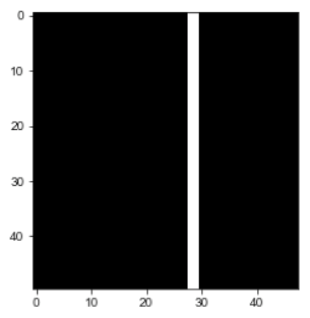### 案例2：图像中物体边缘检测¶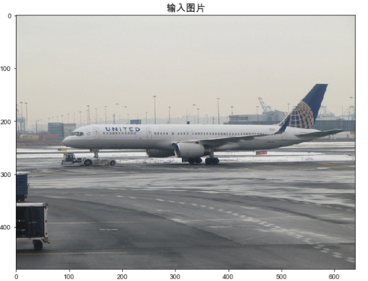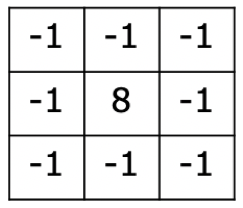import matplotlib.pyplot as plt
from PIL import Image
import numpy as np
img = Image.open('./img/example1.jpg')

# 设置卷积核参数
w = np.array([[-1,-1,-1], [-1,8,-1], [-1,-1,-1]], dtype='float32')/8
w = w.reshape([1, 1, 3, 3])
# 由于输入通道数是3，将卷积核的形状从[1,1,3,3]调整为[1,3,3,3]
w = np.repeat(w, 3, axis=1)
# 创建卷积算子，输出通道数为1，卷积核大小为3x3，
# 并使用上面的设置好的数值作为卷积核权重的初始化参数
conv = Conv2D(in_channels=3, out_channels=1, kernel_size=[3, 3],
initializer=Assign(value=w)))

# 将读入的图片转化为float32类型的numpy.ndarray
x = np.array(img).astype('float32')
# 图片读入成ndarry时，形状是[H, W, 3]，
# 将通道这一维度调整到最前面
x = np.transpose(x, (2,0,1))
# 将数据形状调整为[N, C, H, W]格式
x = x.reshape(1, 3, img.height, img.width)
y = conv(x)
out = y.numpy()
plt.figure(figsize=(20, 10))
f = plt.subplot(121)
f.set_title('input image', fontsize=15)
plt.imshow(img)
f = plt.subplot(122)
f.set_title('output feature map', fontsize=15)
plt.imshow(out.squeeze(), cmap='gray')
plt.show()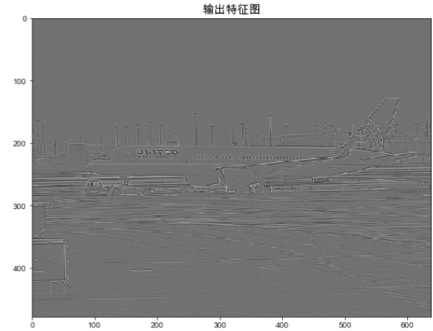### 案例3：图像均值模糊¶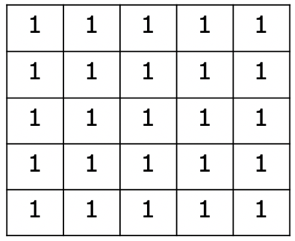import paddle
import matplotlib.pyplot as plt
from PIL import Image
import numpy as np
# 读入图片并转成numpy.ndarray
# 换成灰度图
img = Image.open('./img/example2.jpg').convert('L')
img = np.array(img)

# 创建初始化参数
w = np.ones([1, 1, 5, 5], dtype = 'float32')/25
conv = Conv2D(in_channels=1, out_channels=1, kernel_size=[5, 5],
initializer=Assign(value=w)))
x = img.astype('float32')
x = x.reshape(1,1,img.shape, img.shape)
y = conv(x)
out = y.numpy()

plt.figure(figsize=(20, 12))
f = plt.subplot(121)
f.set_title('input image')
plt.imshow(img, cmap='gray')

f = plt.subplot(122)
f.set_title('output feature map')
out = out.squeeze()
plt.imshow(out, cmap='gray')

plt.show()